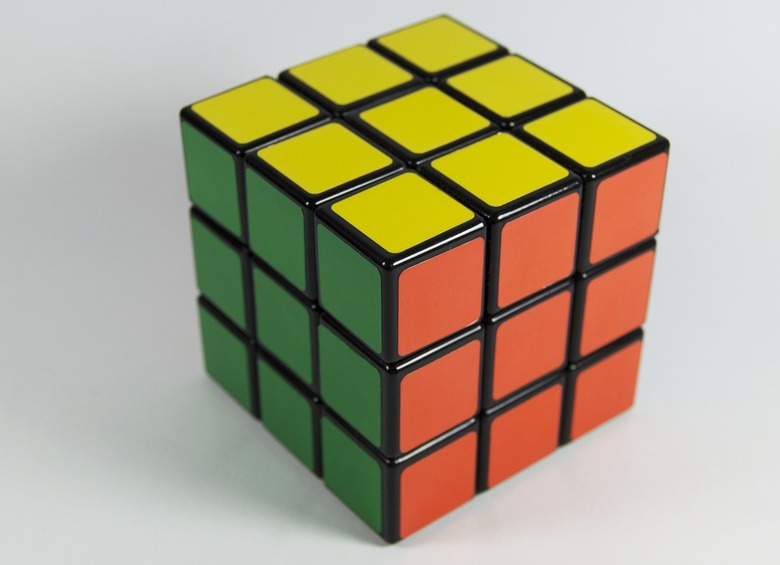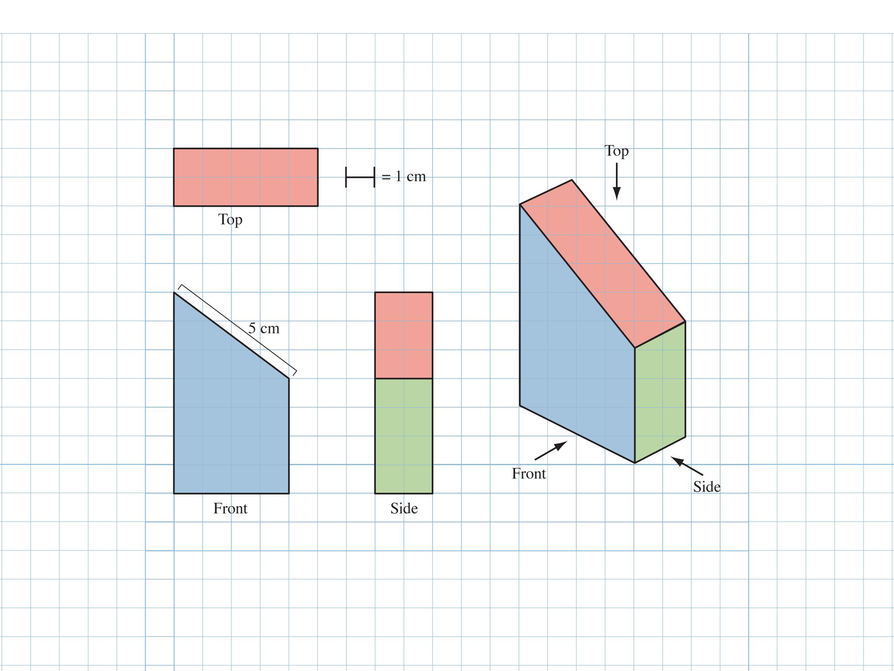Subject:
Geometry
Material Type:
Lesson Plan
Level:
Middle School
7
Provider:
Pearson
Tags:
7th Grade Mathematics, Prisms, Surface Area
Language:
English
Media Formats:
Text/HTML

# Self Check Exercise## Overview

Students will critique their work from the Self Check in the previous lesson and redo the task after receiving feedback. Students will then take a quiz to review the goals of the unit.

# Key Concepts

Students understand how to find the surface area (using nets) and volume of rectangular prisms. They have extended that knowledge to all right prisms and were able to generalize rules for both measurements. Students also found the surface area (and volume) of figures made up of cubes by looking at the 2-D views.

# Goals

• Critique and revise student work.
• Apply skills learned in the unit.
• Understand 3-D measurements:
• Surface area and volume of right prisms
• Area and circumference of circles
• Surface area and volume of figures composed of cubes

SWD: Consider the prerequisite skills for this Putting it Together lesson. Students with disabilities may need direct instruction and/or guided practice with the skills needed to complete the tasks in this lesson. It may be helpful to pull individual students or a small group for direct instruction or guided practice with the skills they have learned thus far in this unit. While students have had multiple exposures to the domain-specific terms, students with disabilities will benefit from repetition and review of these terms. As students move through the lesson, check to ensure they understand the meaning of included domain-specific vocabulary. Use every opportunity to review and reinforce the meaning of domain-specific terms to promote comprehension and recall.

# Lesson Guide

Students should look at the results of their Self Check and think about the critique questions.

# Critique

Review your work on the Self Check problem and think about these questions:

• Can you break up the front surface into shapes that you know how to find the area of?
• What are the length and width of each side?
• What would the opposite side of the figure look like?
• How can you use the area of the front surface to find the volume of the figure?
• Find a different way to solve the problem.# Lesson Guide

Discuss the Math Mission. Students apply what they know about 3-D measurement to find the surface area and volume of a sliced prism.

## Opening

Apply what you know about 3-D measurement to find the surface area and volume of a sliced prism.

# Lesson Guide

Have students work with their partners. Give out a fresh piece of paper to each pair of students. Ask them to try the task again, this time combining their ideas.

# Preparing for Ways of Thinking

As students work with their partners:

• Note different student approaches to the task.
• Note students’ chosen problem-solving approaches.

Attend to these questions:

• Which math do students use?
• Which resources do students ask for?

Attend in particular to  students’ mathematical decisions.

• Do students track their progress in using their chosen mathematics?
• Do students notice if they have chosen a strategy that does not seem to be productive? If so, what do they do?
• Do students use the formulas for surface area and volume correctly?
• Do students draw 2-D views to find the surface area of figures made of cubes?

Try not to make suggestions that move students toward a particular approach to this task. Instead, ask questions that help students clarify their thinking.

If students find it difficult to get started, ask questions such as:

• What do you already know? What do you need to know?
• What is the perimeter of the base? (If you can’t measure exactly, what is a good estimate?) What is its area?
• How many points define a plane?

Look for students who use different methods to answer the questions so that they can be compared during the class discussion.

SWD: Students with disabilities may not readily identify appropriate resources to use as supports. Check to ensure they are aware of the resources available and provide any adaptive tools as necessary.

SWD: Be sure that your pace is appropriate and, if needed at any point, present the questions in writing.

1. The rectangle is 2 cm wide and 5 cm long. It appears to be 4 cm long in the top view because the figure is 4 cm long, and this is how the surface would appear.

2. The surface area is 84 cm${}^{2}$. There are 6 surfaces to account for. The front/back can be broken into a square and a right triangle:

Front/Back: (4 cm ⋅ 4 cm) + (4 cm ⋅ 3 cm)${}^{2}$ = 16 cm${}^{2}$ + 6 cm${}^{2}$ = 22 cm${}^{2}$
22 cm${}^{2}$ ⋅ 2 = 44 cm${}^{2}$

Top: 5 cm ⋅ 2 cm = 10 cm${}^{2}$

Bottom: 4 cm ⋅ 2 cm = 8 cm${}^{2}$

Side: 2 cm ⋅ 4 cm = 8 cm${}^{2}$

Opposite Side: 2 cm ⋅ 7 cm = 14 cm${}^{2}$

Total: 44 cm${}^{2}$ + 10 cm${}^{2}$ + 8 cm${}^{2}$ + 8 cm${}^{2}$ + 14 cm${}^{2}$ = 84 cm${}^{2}$

Alternately, students could look at the figure as a trapezoidal prism and calculate the area of the bases plus the lateral surface area:

Base: 22 cm${}^{2}$

Lateral Surface Area: (5 cm + 4 cm + 4 cm + 7 cm)(2 cm) = (20 cm ⋅ 2 cm) = 40 cm${}^{2}$

Total: 2(22 cm${}^{2}$) + 40 cm${}^{2}$ = 44 cm${}^{2}$ + 40 cm${}^{2}$ = 84 cm${}^{2}$

3. The volume is 44 cm${}^{3}$. If the trapezoid is considered the base (whose area is 22 cm${}^{2}$), then that area can be multiplied by the “height” (2 cm):

22 cm${}^{2}$ ⋅ 2 cm = 44 cm${}^{3}$

The figure could also be broken up into two parts—a rectangular prism and a half-rectangular prism: (4 cm ⋅ 4 cm ⋅ 2 cm) + (4 cm ⋅ 3 cm ⋅ 2 cm${}^{2}$) = 32 cm${}^{3}$ + 12 cm${}^{3}$ = 44 cm${}^{3}$

# Revise and Extend Your Work

Work with your partner to revise your work on the Self Check problem based on the previous questions and feedback from your partner.

Self Check Problem

Use the drawings to answer the following questions. Note that the surface areas of the top and bottom surfaces will not be the same because the prism has been sliced. For the same reason, the surface areas of the side surfaces will be different.

1. What is the length and width of the rectangle cross section revealed?
2. What is the surface area of the figure? Show how you found your answer.
3. What is the volume of the figure? Show how you found your answer.# Challenge Problem

Create a 2-D view of a 3-D figure on paper, and give it to your partner to create the 3-D figure in the 3-D builder. (Have your partner ignore the figure shown at the bottom-right).

INTERACTIVE: 3-D Builder

# Lesson Guide

Organize a whole class discussion to consider issues arising from the work students did to revise their work. You may not have time to address all these issues, so focus the class’s discussion on the issues most important for students.

Have students share their work and talk about how they approached the problem. Students with strategies that didn’t work should share so they can talk about how and when they realized their strategy didn’t work and what they did about it. Have students share the Self Check assessment questions (from the teacher or generated by the system) that they addressed and how they addressed those questions. Have students ask questions and make observations as they view each other’s work.

• What part of the task was most difficult?
• Did you and your partner ever disagree about measurements? Did you resolve your difference of opinion? How?

SWD: This is an excellent opportunity to support students with disabilities who may have difficulty with cooperative learning tasks. If students have trouble resolving conflicts and/or disagreements, coach in to help students recognize the importance of considering alternate perspectives. Also, provide suggestions as to how students can navigate differences of opinion in learning situations.

ELL: In forming groups, be aware of your ELLs and ensure that they have a learning environment where they can be productive. Sometimes, this means pairing them up with English speakers from whom they can learn language skills. Other times, it means pairing them up with students who are at the same level of language skill, which allows them to take a more active role and work things out together. Yet, other times, it means pairing them up with students whose proficiency level is lower, so they play the role of the supporter. You can also pair students based on their math proficiency, not just their language proficiency.

# Ways of Thinking: Make Connections

Take notes about your classmates’ approaches to revising and solving the Self Check problem.

## Hint:

• What part of the task was most difficult?
• What errors in your work did you have to revise? How did you go about revising the mistakes?
• Did you and your partner ever disagree about some aspect of the problem? If so, were you able to resolve your difference of opinion? How?
• How did you use the two-dimensional views of the figure to help you find the surface area and volume of the figure?
• What shapes (or figures) did you break the front view into to help you find the surface area (or volume)?
• Did you find two ways to solve the problem? Can you explain what they are?

# Lesson Guide

Have each student write a brief reflection before the end of class. Review the reflections to see how students apply what they know about 3-D measurement to find the surface area and volume of a sliced prism. If you notice insightful comments, plan to share them with the class in the next lesson.

ELL: When writing the reflection, allow some additional time for ELLs to discuss with a partner before writing, to help them organize their thoughts. Allow ELLs who share the same primary language to discuss in their preferred language, if they wish, and to use a dictionary.

# Reflection

Write a reflection about the ideas discussed in class today. Use the sentence starter below if you find it to be helpful.

Some things I learned in this unit about 3-D figures are …